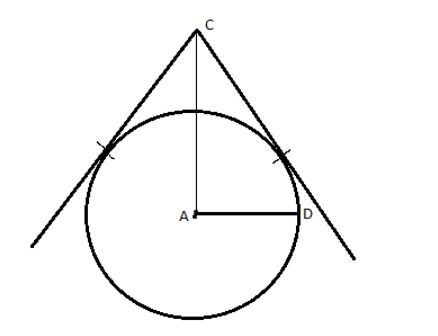Courses
Courses for Kids
Free study material
Free LIVE classes
MoreLIVE
Join Vedantu’s FREE Mastercalss

# Draw a circle of radius 3 cm and construct two tangents to it from an external point 8 cm away from its center.Verified
335.1k+ views
Hint: In order to construct a circle of radius and two tangents from it, we make a construction using the appropriate geometrical equipment. We make use of a ruler to measure the length of the radius and an external point 8 cm from the center of the circle. Then we draw two lines to the circle from the external point such that thy touch the circle at exactly one point respectively.

Given Data:
Radius of the circle = 3 cm
Length of the external point from the center of circle = 8 cm

The steps of construction to construct the circle and tangents to it are as follows:
First we draw a line segment of length 3 cm and name it ‘AD’, which acts as the radius of the circle.
Then with point A as the center of the circle we draw a full arc starting from point B as the other end until we make a full circle and reach point B again.
Now from point A we measure a length of 8 cm towards any direction of our choice and mark a point C there.
Now with C as the starting point we draw a line towards any edge of the circle such that it only touches the circle at one point throughout its entire course.
Now with C as the center, we repeat the same process but this time we draw the line in a different direction from the previous.
Thus we made a circle with two tangents to it from an external point.
Thus the circle with center A is obtained.
The figure obtained after we finish construction is as follows:Note: In order to solve this type of problems the key is to know how to construct a geometrical figure with a set of given requirements, using the help of geometrical apparatus. We measure a length of straight lines using a ruler, we draw the circle using a compass to which one end is fixed at the center of circle A and the other end is placed at the point B and is freely moved in a circular direction until we reach B again. We find all these instruments in a geometrical box and each instrument has a specific use case. We do the construction in a stepwise manner.
Last updated date: 28th Sep 2023
Total views: 335.1k
Views today: 10.35k